Back to Adaptive Vision Library website

You are here: Start » Function Reference » Geometry 2D Features

# Geometry 2D Features

Select a function from the list below.

IconNameDescription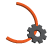ArcCircleComputes the circle containing an arc.ArcEndpointsComputes two endpoints of an arc.ArcLengthComputes the length of an arc.ArcMidpointComputes the midpoint of an arc.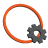CircleAreaComputes the area of a circle.CircleBoundingBoxComputes the smallest box containing a circle.CircleBoundingRectangleComputes the smallest rectangle containing a circle.CircleCharacteristicPointReturns a characteristic point (e.g. the top-left) of a box containing the input circle.CirclePerimeterLengthComputes the length of a circle perimeter.CircleSectionComputes an arciform section of the circle perimeter.LineNormalVectorComputes the normal vector of a lineLineOrientationComputes the orientation angle of a line as angle in the range from 0 to 180.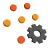PointsBoundingBoxComputes the smallest box containing an array of points.PointsBoundingBox_OrNilComputes the smallest box containing an array of points; returns NIL if the array is empty.PointsBoundingCircleComputes the smallest circle containing an array of points.PointsBoundingCircle_OrNilComputes the smallest circle containing an array of points; returns NIL if the array is empty.PointsBoundingParallelogramComputes the smallest parallelogram containing an array of points.PointsBoundingRectangleComputes the smallest rectangle containing an array of points.PointsBoundingRectangle_FixedAngleComputes the smallest rectangle with the given orientation angle containing an array of points.PointsBoundingRectangle_FixedAngle_OrNilComputes the smallest rectangle with the given orientation angle containing an array of points; returns NIL when the array is empty.PointsBoundingRectangle_OrNilComputes the smallest rectangle containing an array of points; returns NIL when the array is empty.PointsConvexHullComputes the smallest convex shape that contains the given array of points.PointsDiameterFinds the longest segment connecting two points from a given array.PointsMassCenterComputes the central point of the input points.PointsMassCenter_OrNilComputes the central point of the input points; returns NIL if the array is empty.PointsMedianComputes the geometric median of the input points.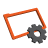RectangleAreaComputes the area of a rectangle.RectangleBoundingBoxComputes the smallest box containing a rectangle.RectangleBoundingCircleComputes the smallest circle containing a rectangle.RectangleCenterComputes the center point of a rectangle.RectangleCharacteristicPointReturns a characteristic point (e.g. the top-left) of the input rectangle.RectangleCharacteristicPointsComputes rectangle's characteristic points.RectangleCornersComputes the four corner points of a rectangle.RectanglePerimeterLengthComputes the perimeter length of a rectangle.SegmentBisectorComputes a line passing through the center of a segment at a right angle.SegmentCenterComputes the center point of a segment.SegmentLengthComputes the length of a segment.SegmentLineComputes the line passing through a segment.SegmentNormalVectorComputes an unitary vector perpendicular to the segment.SegmentOrientationComputes the orientation of a segment.SegmentVectorReturns the vector [x2 - x1, y2 - y1]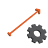VectorDirectionComputes the direction angle of a vector as an angle in range the from 0 to 360.VectorLengthComputes the length of a vector.VectorsMedianComputes the geometric median of the input vectors.